Home MonkeyNotes Printable Notes Digital Library Study Guides Message Boards Study Smart Parents Tips College Planning Test Prep Fun Zone Help / FAQ How to Cite New Title Request

 Example 1 Areas of two similar triangles are 144 sq.cm. and 81 sq.cm. If one side of the first triangle is 6 cm then find the corresponding side of the second triangle. Solution :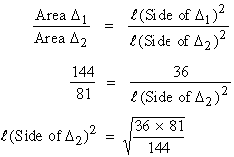= 4.5 c.m. Example 2 The side of an equilateral triangle ABC is 5 cm. Find the length of the side of another equilateral D PQR whose area is four times area of D ABC. Solution: Since both triangles are equilateral they are similar.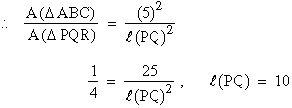Example 3 The corresponding sides of two similar triangles are 4 cm and 6 cm. Find the ratio of the areas of the triangles. Solution: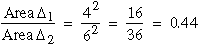Example 4 D ABC ~ D PQR such that l (seg.AB) : l (seg.PQ) that is 8 : 6. If area of D ABC is 48 sq.cm what is the area of the smaller triangle. Solution: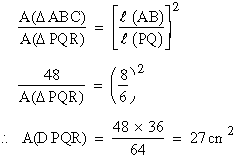Example 5 In a trapezium ABCD, side AB çç CD. The diagonals AC and BD cut each other at M. Prove that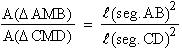Solution: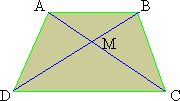To Prove thatConsider D AMB and D CMD. Ð AMB @ Ð CMD, vertical angles Ð BAM @ Ð DCM alternate angles \ By AA test D AMB ~ D CMD \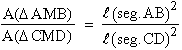********** Index 5.1 Introduction 5.2 Ratio And Proportionality 5.3 Similar Polygons 5.4 Basic Proportionality Theorem 5.5 Angle Bisector Theorem 5.6 Similar Triangles 5.7 Properties Of Similar Triangles
 Search: All Products Books Popular Music Classical Music Video DVD Toys & Games Electronics Software Tools & Hardware Outdoor Living Kitchen & Housewares Camera & Photo Cell Phones Keywords: